• huberloss

2018-10-13 21:09:44
huber loss huber loss 是一种优化平方loss的一种方式，使得loss变化没有那么大。 import numpy as np import matplotlib.pyplot as plt import tensorflow as tf def huber_loss(x, d): x = np.abs(x) return ...

huber loss

huber loss 是一种优化平方loss的一种方式，使得loss变化没有那么大。

import numpy as np
import matplotlib.pyplot as plt
import tensorflow as tf

def huber_loss(x, d):
x = np.abs(x)
return ( x<=d ) * x**2 /2 + ( x>d ) * d *( x - d /2.0)

# tf.losses.huber_loss()

if __name__ == '__main__':
plt.figure(figsize=(6, 4.5), facecolor='w', edgecolor='k')
x = np.linspace(-20, 20, 10000)
plt.plot(x, x** 2 / 2, label='squared loss')
for d in (10, 5, 3, 1):
plt.plot(x, huber_loss(x, d), label=r'huber loss: $\delta$={}'.format(d))
plt.legend(loc='best', frameon=False)
plt.xlabel('residual')
plt.ylabel('loss')
plt.show()

显示的图像如下：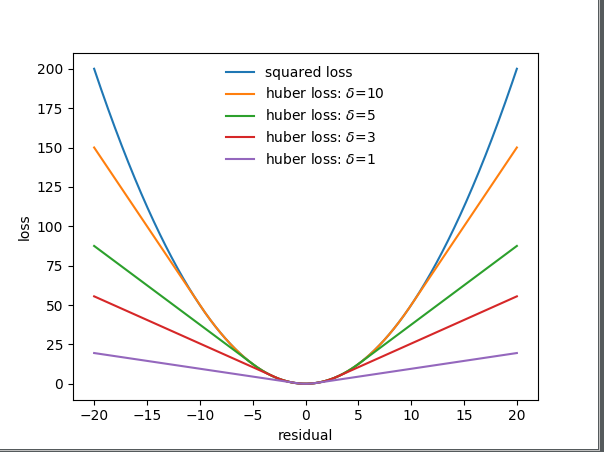展开全文huber loss
• 常用loss： L1： 公式：L1=∑i=1n∣yi−f(xi)∣L1=\sum_{i=1}^{n}\left|y_{i}-f\left(x_{i}\right)\right|L1=i=1∑n​∣yi​−f(xi​)∣ 导数：dL1(x)dx={1 if x≥0−1 otherwise \frac{\...

常用loss：

L1：

• 公式： L 1 = ∑ i = 1 n ∣ y i − f ( x i ) ∣ L1=\sum_{i=1}^{n}\left|y_{i}-f\left(x_{i}\right)\right|
• 导数： d L 1 ( x ) d x = { 1  if  x ≥ 0 − 1  otherwise  \frac{\mathrm{d} L_{1}(x)}{\mathrm{d} x}=\left\{\begin{array}{ll}{1} & {\text { if } x \geq 0} \\ {-1} & {\text { otherwise }}\end{array}\right.

L2：

• 公式： L 2 = ∑ i = 1 n ( y i − f ( x i ) ) 2 L2=\sum_{i=1}^{n}\left(y_{i}-f\left(x_{i}\right)\right)^{2}
• 导数： d L 2 ( x ) d x = 2 x \frac{\mathrm{d} L_{2}(x)}{\mathrm{d} x}=2 x
• 特性：对离群点比较敏感，需要自习调整学习率，防止出现梯度爆炸的情况(因为两端值很大)；

smooth L1：

• 公式： smooth ⁡ L 1 ( x ) = { 0.5 x 2  if  ∣ x ∣ < 1 ∣ x ∣ − 0.5  otherwise  \operatorname{smooth}_{L_{1}}(x)=\left\{\begin{array}{ll}{0.5 x^{2}} & {\text { if }|x|<1} \\ {|x|-0.5} & {\text { otherwise }}\end{array}\right.
• 导数： d smooth ⁡ L 1 d x = { x  if  ∣ x ∣ < 1 ± 1  otherwise  \frac{\mathrm{d} \operatorname{smooth}_{L_{1}}}{\mathrm{d} x}=\left\{\begin{array}{ll}{x} & {\text { if }|x|<1} \\ { \pm 1} & {\text { otherwise }}\end{array}\right.

Huber loss：

• 公式： L δ ( y , f ( x ) ) = { 1 2 ( y − f ( x ) ) 2 ,  for  ∣ y − f ( x ) ∣ ≤ δ δ ⋅ ( ∣ y − f ( x ) ∣ − 1 2 δ ) ,  otherwise  L_{\delta}(y, f(x))=\left\{\begin{array}{ll}{\frac{1}{2}(y-f(x))^{2},} & {\text { for }|y-f(x)| \leq \delta} \\ {\delta \cdot\left(|y-f(x)|-\frac{1}{2} \delta\right),} & {\text { otherwise }}\end{array}\right.
• 优点是能增强平方误差损失函数(MSE)对离群点的鲁棒性，用于回归问题。δ是一个可以自己设置的参数。
当预测偏差小于 δ 时，它采用平方误差,
当预测偏差大于 δ 时，采用的线性误差。
[外链图片转存失败(img-TExue3Iw-1568215766580)(./1567858938658.png)]
如上图绿色部分为huber loss。
[外链图片转存失败(img-kAJeSxmv-1568215766580)(./1567846985700.png)]

说明

L1loss在零点不平滑，学习慢；
L2loss学习快，因为是平方增长，但是当预测值太大的时候，会在loss中占据主导位置(如真实值为1，预测多次，有一次预测值为100，其余预测为2)；
Smooth L1 Loss 相比L1修改零点不平滑问题，而且在x较大的时候不像L2对异常值敏感，是一个缓慢变化的loss；
Huber loss增强L2对离群点的鲁棒性，因为偏差大的时候变成线性增长；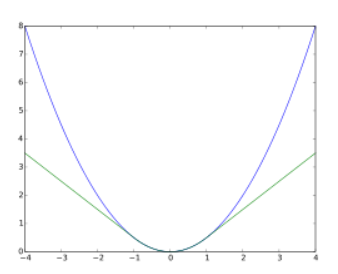上图绿色部分为huber loss，紫色部分为L2loss；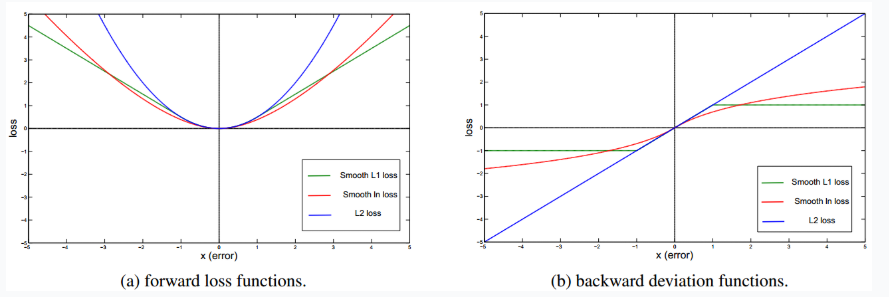上图为smooth L1 loss和L2 loss的导数对比，在两端smooth L1 导数恒定为1，而L2 loss会一直上升；

另外L1和L2范数常用于正则化项：

L1正则会制造稀疏的特征，大部分无用特征的权重会被置为0，有特征选择作用；
L2正则会让特征的权重不过大，使得特征的权重比较平均。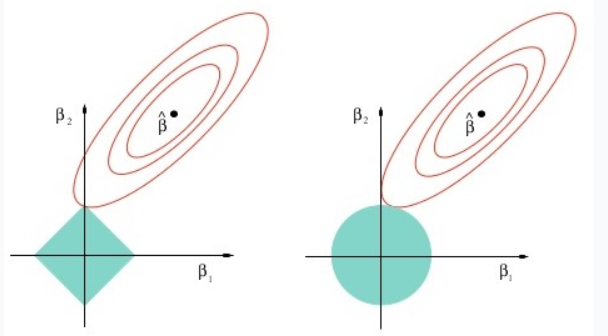上图为1范数和2范数的图像，可以看出L1正则倾向于选择坐标轴上的参数（即出现0为稀疏解），L2正则倾向于选择均匀参数；

展开全文loss 深度学习 机器学习
• 机器学习之Huberloss

千次阅读 2020-04-03 20:12:34
Huber Loss 是用于回归问题的带参损失函数, 优点是能增强平方误差损失函数(MSE, mean square error)对离群点的鲁棒性。 当预测偏差小于 δ 时，它采用平方误差, 当预测偏差大于 δ 时，采用的线性误差。 具体定义...

Huber Loss 是用于回归问题的带参损失函数, 优点是能增强平方误差损失函数(MSE, mean square error)对离群点的鲁棒性。

• 当预测偏差小于 δ 时，它采用平方误差,
• 当预测偏差大于 δ 时，采用的线性误差。

具体定义如下：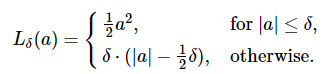其中 a 表示误差，即 y − y ^ y-\hat{y} 或者 y − f ( x ) y-f(x) ，因此上式可变为：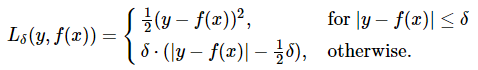示例如下：

import numpy as np
import matplotlib.pyplot as plt

def huber_loss(a, d):                    # Definition
return (abs(a)<=d)*a**2/2 + (abs(a)>d)*d*(abs(a)-d/2)

plt.figure(figsize=(6, 4.5), facecolor='w', edgecolor='k')

x = np.arange(-30, 30)
plt.plot(x, x**2/2, lw=2,label='Squared loss', color='g')
for d in (10, 6, 3, 1):
plt.plot(x, huber_loss(x, d), label=r'Huber loss: $\delta$={}'.format(d), lw=2)

plt.legend(loc='best', frameon=False)
plt.xlabel('standard deviation')
plt.ylabel('loss')
plt.show()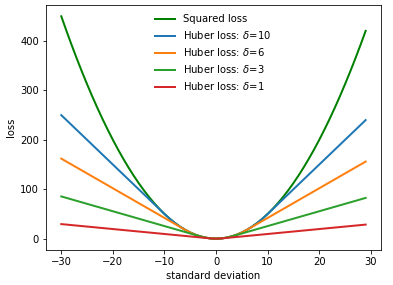δ 是 HuberLoss 的参数，y 是真实值，f(x) 是模型的预测值, 横坐标 x 则是误差值。

Reference：

展开全文• Huberloss

2017-04-26 17:54:00
统计学中，Huber损失是用于鲁棒回归的损失函数，与平方误差损失相比，对数据中的游离点较不敏感。 也有时使用分类的变体。 1.定义 胡伯损失函数描述估计方法F招致的惩罚。Huber（1964）通过分段定义了损失函数。 当...

统计学中，Huber损失是用于鲁棒回归的损失函数，与平方误差损失相比，对数据中的游离点较不敏感。 也有时使用分类的变体。

1.定义

胡伯损失函数描述估计方法F招致的惩罚。Huber（1964）通过分段定义了损失函数。当a的值较小时，该函数为二次函数，当a的值较大时，该函数为线性函数，当|a|=delta时两函数具有相等的值和不同的斜率。变量a通常是指残差，即观测值和预测值之间的差值a=y-f(x)。所以上面的式子可以被扩展为：两个非常常用的损失函数是平方丢失，L（a）= a ^ 2，和绝对损失L（a）= | a |。 平方损失函数导致算术平均无偏估计，并且绝对值损失函数导致中值无偏估计量（在一维情况下，多维情况下的几何中位数 - 无偏估计量）。 平方损失的缺点是，它具有被异常值支配的倾向 - 当对一组a进行求和时（如L（a1）+...L(an).当分布很重时，样本平均值受到一些特别大的a值的影响太大.：在估计理论方面，平均值的渐近相对效率对于重尾分布是很差。
如上所述，Huber损失函数在其最小值a = 0的均匀邻域中是凸的，在该均匀邻域的边界处，Huber损失函数在点处具有可微分的仿射函数的扩展a=-delta和 a=delta。 这些属性允许它将平均无偏差的最小方差估计器（使用二次损耗函数）和中值无偏估计器的鲁棒性（使用绝对值函数）的大部分灵敏度相结合。

2.Pseudo-Huber loss 函数

Pseudo-Huber loss 函数可以用作Huber loss 函数的平滑近似，并确保派生物在所有程度上是连续的。 它被定义为因此，对于a的小值，该函数近似于a ^ 2 / 2， 对于a的大值该函数近似于具有斜率delta。
虽然上述是最常见的形式，但是还存在Huber损失函数的其他平滑近似

3.用于分类的变体

为了分类，有时使用Huber损失的变体。 给定预测值f（x）（一个实值分类器得分）和一个真正的二进制类标签 y in (+ 1，-1)，修改后的Huber损失定义为术语max（0,1-y.(f（x)^2)）是支持向量机使用的铰链损失; 二次平滑的铰链损失L的泛化

4.应用

Huber损失函数用于鲁棒统计，M估计和加法建模

转载于:https://www.cnblogs.com/bbn0111/p/6769876.html

展开全文• P s e u d o _ h u b e r _ l o s s = δ 2 ( 1 + ( y ^ − y δ ) 2 + 1 ) Pseudo\_huber\_loss= \delta ^2(\sqrt{1 + (\frac{\hat{y} - y}{\delta})^2} + 1) Pseudo_huber_loss=δ2(1+(δy^​−y​)2 ​+1) 一阶...机器学习 python 人工智能
• 【损失函数】MSE, MAE, Huberloss详解

千次阅读 多人点赞 2020-12-15 19:51:59
...wfr=spider&for=pc 无论在机器学习还是深度领域中,损失函数都是一个...损失函数（Loss Function）是用来估量模型的预测值 f(x) 与真实值 y 的不一致程度。我们的目标就是最小化损失函数，让 f(x) 与 y 尽量接...
• HuberLoss

千次阅读 2019-08-05 17:01:19
Huber Loss 是一个用于回归问题的带参损失函数, 优点是能增强平方误差...相比于最小二乘的线性回归，HuberLoss降低了对离群点的惩罚程度，所以 HuberLoss 是一种常用的鲁棒的回归损失函数。 Huber Loss 定义如下:...机器学习 Huber
• HuberLoss 介绍

2019-10-03 15:57:45
Huber Loss 是一个用于回归问题的带参损失函数, 优点是能增强平方误差损失函数(MSE,...相比于最小二乘的线性回归，HuberLoss降低了对离群点的惩罚程度，所以 HuberLoss 是一种常用的鲁棒的回归损失函数。 Huber Los...
• Huber Loss Log-Cosh Loss Quantile Loss Huber Loss Huber损失函数（ Smooth L 1 \text{Smooth} L_1 Smooth L 1 ​ 损失函数是其的一个特例）整合了MAE和MSE各自的优点，并避免其缺点 L δ ( y , f ( x...
• 回归损失函数：HuberLoss

万次阅读 多人点赞 2019-05-07 16:17:12
Huber损失函数，平滑平均绝对误差 相比平方误差损失，Huber损失对于数据中异常值的敏感性要差一些。在值为0时，它也是可微分的。它基本上是绝对值，在误差很小时会变为平方值。误差使其平方值的大小如何取决于一个...回归 Huber Loss
• HuberLoss function

2019-02-22 21:08:56
转自：https://blog.csdn.net/lanchunhui/article/details/50427055
• HuberLoss 及 tensorflow实现

千次阅读 2018-03-15 17:22:33
Huber Loss 相当于平方误差的推广，通过设置delta的值，使损失函数鲁棒性更强，从而减弱离群点（outliers）对模型的影响。当delta为无穷大时，Huber Loss 退化为Squared Loss. 解释： Huber Loss对平方损失进行...
• Huberloss function

2019-07-17 17:27:15
中间是二次函数，两侧是线性函数。 Manipulate[Plot[Piecewise[{{x^2/2, Abs[x] < d}, {d (Abs[x] - d/2), Abs[x] > d}}], {x, -10, 10}, PlotRange -> {{-10, 10}, {-1, 40}}], {d, 0, 10, 0.1}] ...
• Huber Loss Log-Cosh Loss Quantile Loss Huber Loss Huber损失函数（SmoothL1SmoothL1损失函数是其的一个特例）整合了MAE和MSE各自的优点，并避免其缺点   Lδ(y,f(x))={12(y−f(x))2δ∣y−f(x)...
• AttributeError: module 'torch.nn' has no attribute 'HuberLoss'深度学习 pytorch 神经网络
• MAE、MSE、Huberloss详解

千次阅读 2018-11-05 18:47:54
https://mp.weixin.qq.com/s/Xbi5iOh3xoBIK5kVmqbKYA
• Huberloss--转

2019-09-27 03:41:24
原文地址：https://en.wikipedia.org/wiki/Huber_loss ...Instatistics, theHuber lossis aloss functionused inrobust regression, that is less sensitive tooutliersin data than thesquared error loss. ...
• 再试一种Huber损失、或者Tukey损失函数最小二乘回归 from sklearn . linear_model import HuberRegressor huber = HuberRegressor ( ) . fit ( x , y ) p = huber . predict ( x ) plt . scatter ( x ...
• 【机器学习】Huberloss

千次阅读 2019-08-02 09:51:17
Huber Loss 是一个用于回归问题的带参损失函数, 优点是能增强平方误差损失函数(MSE, mean square error)对噪声（或叫离群点，outliers）的鲁棒性。 当预测偏差小于 δ 时，它采用平方误差, 当预测偏差大于 δ 时，...
• 线性拟合——从最大似然估计到平方误差到huber loss
• 在监督式机器学习中，无论是回归问题还是分类问题，都少不了使用损失函数（Loss Function）。**损失函数（Loss Function）**是用来估量模型的预测值 f(x) 与真实值 y 的不一致程度。若损失函数很小，表明机器学习...机器学习 深度学习 神经网络...